# Magic Math

One of the reasons I love Magic is due to its surprising connections to deep mathematical concepts. (Richard Garfield is a mathematician after all.) So, in the spirit of Quandrix, here are some examples that I hope that you find as interesting as I do.

#### Arithmetic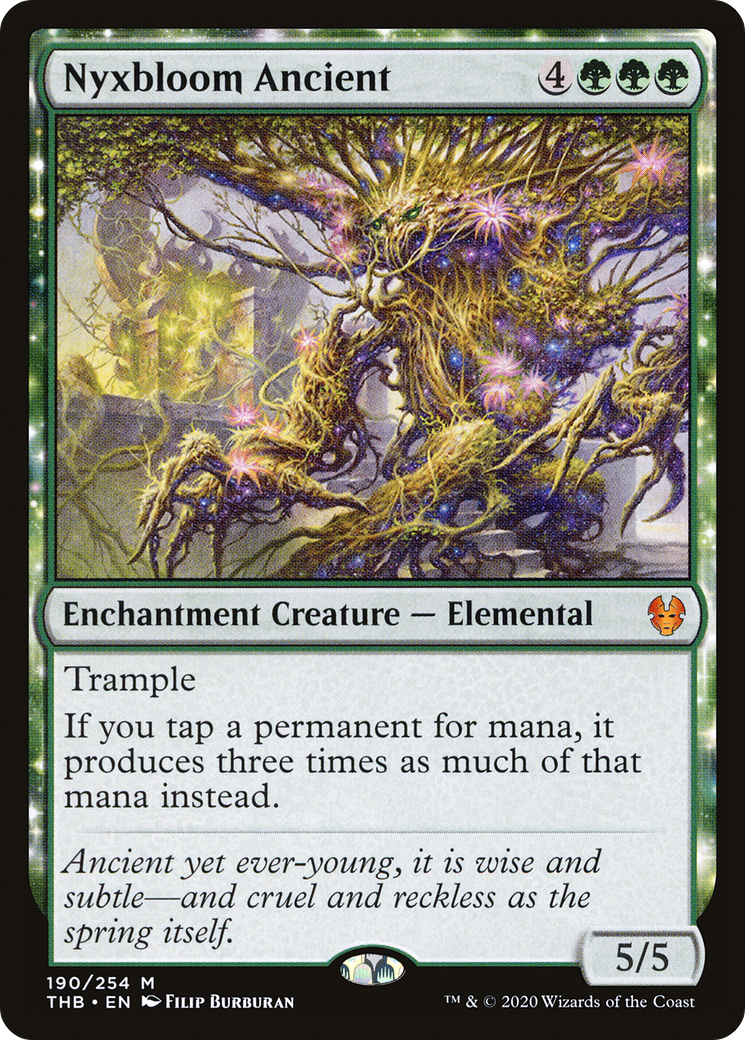Arithmetic is generally the first area of math taught in schools, and consequentially it's often thought of as tedious or dry. Honestly, I kind of agree; anything a calculator can do has got to be kind of boring, and there are far more interesting things to think about. However, arithmetic is probably the most important mathematical skill in our everyday lives, and Magic can do a great job of helping us get more familiar with it.

For example, Wizards loves printing cards that double things. More recently, they've also started tripling things. While pretty straightforward individually, combining these sorts of cards can be a handy playground for people to practice their arithmetic skills and gain a more intuitive understanding of concepts like exponentiation.

If you kick a Rite of Replication targeting Nyxbloom Ancient (for a total of 6 including the tokens and the original) and also control Mana Reflection, how much mana does your Forest tap for? Calculating this by hand is difficult, and don't we get to choose in what order to apply the different replacement effects, making this even more complicated? This becomes easy to solve when we consider what Nyxbloom Ancient actually does; it multiplies the amount of mana the Forest is producing by three. Since multiplication is commutative, we know that the order we apply the effects in won't change the final result, and calculating it is simple: 1⋅3⋅3⋅3⋅3⋅3⋅3⋅2. Or to write that more succinctly, 2⋅(3^6), for a total of 1458 mana from one Forest.

#### Probability Theory

Let's try to figure out how Krark's Thumb changes the probabilities of flipping a coin. I'm going to assume that we always want coins to come up heads, and always choose to ignore tails for Krark's Thumb if possible. Without any Krark's Thumbs, the chance that I win a coin flip is just 1/2. If I control a Krark's Thumb, then I have two chances to win. In probability theory this means that I multiply together the chances of losing and get 1/4, for a 3/4 chance of winning. There's an easier way to see this though. There are four possible results of flipping two coins: both heads, both tails, first one heads and second one tails, or first tails and second heads. As long as there's at least one that comes up heads, I can just choose that one, so the only result where I lose is the tails-tails one, and I win the other 3.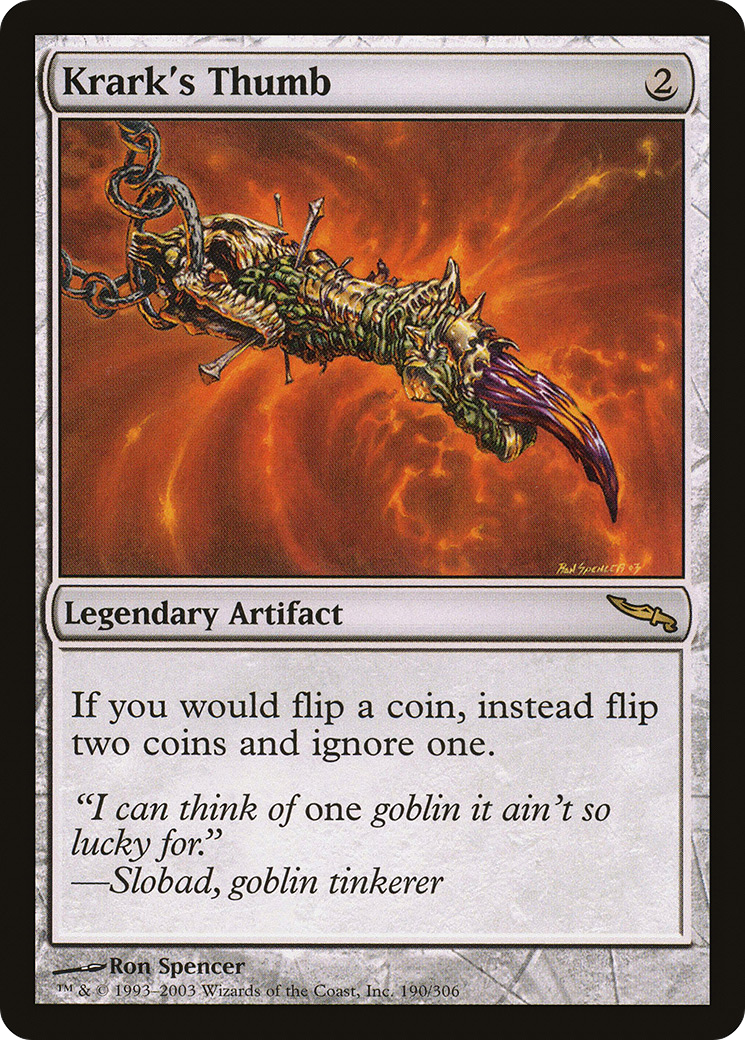Ok, this seems simple enough; it's just powers of 2, right? 1/2, 1/4, 1/8, etc. To turn this into a precise formula, it would mean that the chance I lose is 1 out of 2^N, where N is the number of Krark's Thumbs I have plus one. With no Krark's Thumbs that's 1/2^1, which is 1/2. With one Krark's Thumb that's 1/2^2, which is 1/4. Etc. This is kind of weird though, where's that "plus one" coming from? There doesn't seem like a good reason we'd need to be performing any addition here. Let's check our work. When I have two Krark's Thumbs, I effectively flip 4 coins instead of 1, and can pick my result. So in order to lose, all 4 coins need to come up tails. There are 16 possible results:

• HHHH
• HHHT
• HHTH
• HHTT
• HTHH
• HTHT
• HTTH
• HTTT
• THHH
• THHT
• THTH
• THTT
• TTHH
• TTHT
• TTTH
• TTTT

Only one of these sequences has no heads, so the chance that we lose this flip is actually 1/16, not 1/8. Hmm, where'd we go wrong? Turns out our generalization was faulty. We just saw a pattern (2 followed by 4) and assumed that this was matching the powers of two, without a good reason to believe that. We confused the number of coins we're flipping with the chance that all of those coins come up tails. To figure out the correct formula, we first need to figure out how many coins we're flipping. We start with one coin flip, and each Krark's Thumb doubles the number of coins we flip, so we flip 2^N coins, where N is the number of Krark's Thumbs we have. (If we have no Krark's Thumbs this is still correct, because 2^0=1.) Given some number of coin flips, the chance that all of them come up tails is just equal to the chances that any individual one of them comes up tails multiplied together. For example if I flip 3 coins, the chance that they all come up tails is 2·2·2 = 2^3 = 8. We can see why this is from our earlier list of results; each letter denotes a specific coin. There are two options for the first coin, two options for the second coin, etc. Putting this all together, the correct formula for the chance we flip all tails and lose the flip is 1/2^2^N, where N is the number of Krark's Thumbs. This grows much faster. By the time we have 4 Krark's Thumbs on the battlefield, you can flip 10 thousand coins and on average you'll win every single one. (I'm not sure how Krark managed to lose that many thumbs, but it's clear he would have had to be pretty clumsy.) A neat tool for calculating coin flips is here.

#### Geometry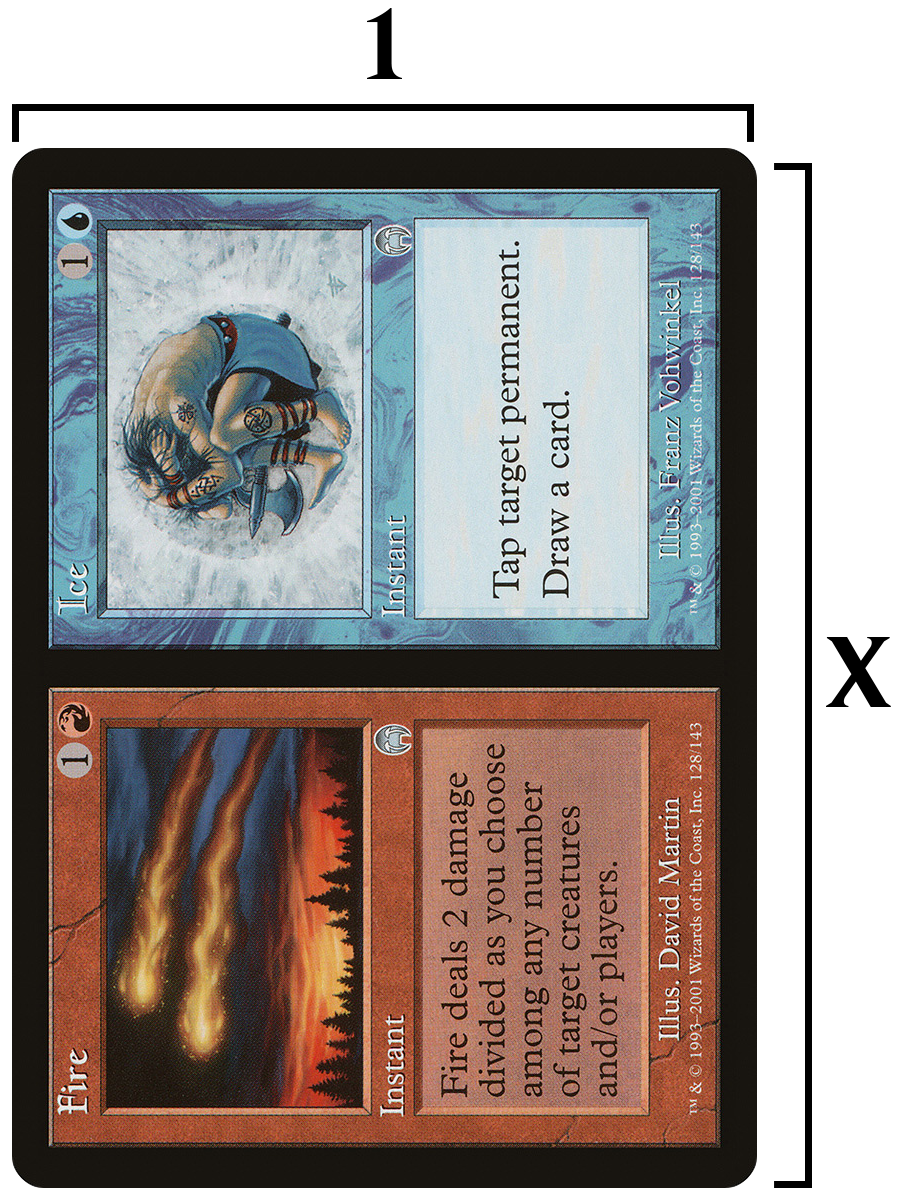Split cards have two tiny cards on one. But wait a minute; each tiny card is the same shape as a regular card. And this relationship holds in the other direction too; oversized commanders are exactly twice as big as a regular card, and the same shape. It's easy to see that quality wouldn't work if Magic cards were square, or if they were really long and thin. There must be a special kind of rectangle that allows this to work. So what exactly is going on here?

Well, this immediately reminds me of the golden ratio. A rectangle with an aspect ratio of the golden ratio can be divided into a square and another smaller rectangle of the exact same shape. This is kinda similar to what we see with split cards, but not quite. We don't want to end up with a square along with our rectangle, we want to end up with two rectangles that are each the same shape as the one we started with. So how do we figure out what ratio this is? Let's put it into algebraic terms and say that shorter edge of the card has length 1, and the longer edge has length X. Then the ratio X/1 (long side to short side) must equal 1/(X/2) (short side to half of the long side). At this point we can just let WolframAlpha do the work for us, and the answer is √2! How neat is that‽ The ratio that Magic cards use approximates an important irrational constant!

Another way to see why √2 has this property is that 1 · √2 = √2, and √2 · √2 = 2, which is twice 1. We can verify this by actually looking at the dimensions of a card, which gives us a ratio of ~1.39, which is pretty close to √2. (~1.41). Wizards would have had to fudge it a little bit on the split cards due to that ~0.02 difference, but the borders are going to have more of an effect anyway (since they have to be constant width on all sides). I measured a split card and the ratio of each tiny card was ~1.46.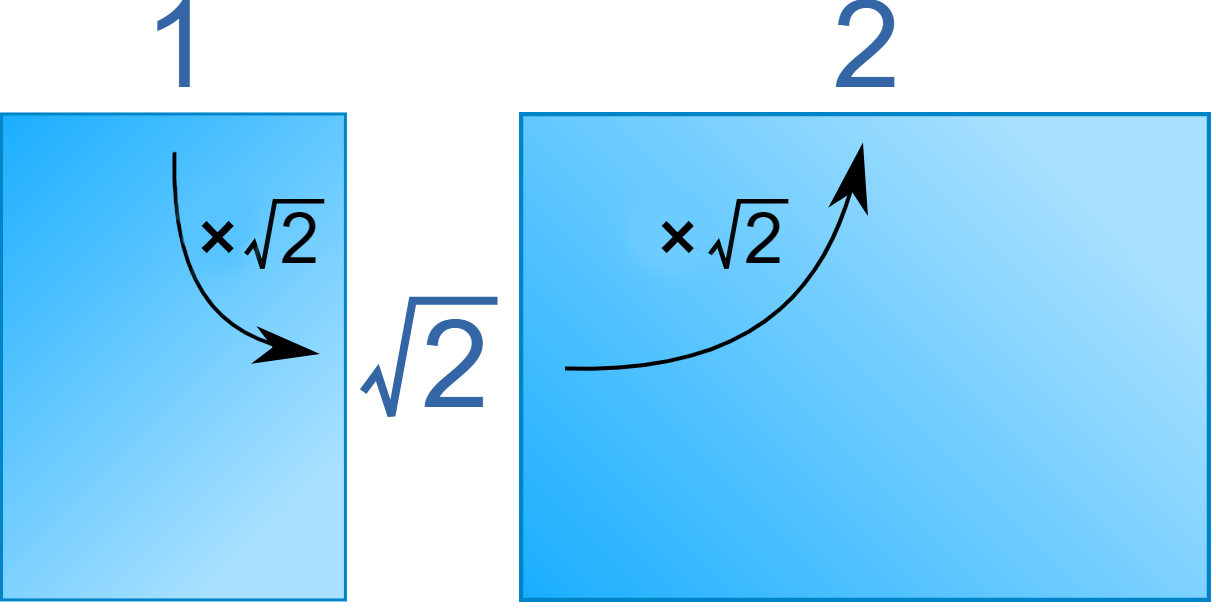Interestingly, the civilized world uses standardized paper sizes that also conform to this ratio. An A5 sheet of paper is half the area of an A4 sheet, which is half of an A3 sheet, while all being the same shape. (Illustration) This makes scaling print material for different usages such as posters and pamphlets nice and easy.

#### Digits, Numerals, and Numbers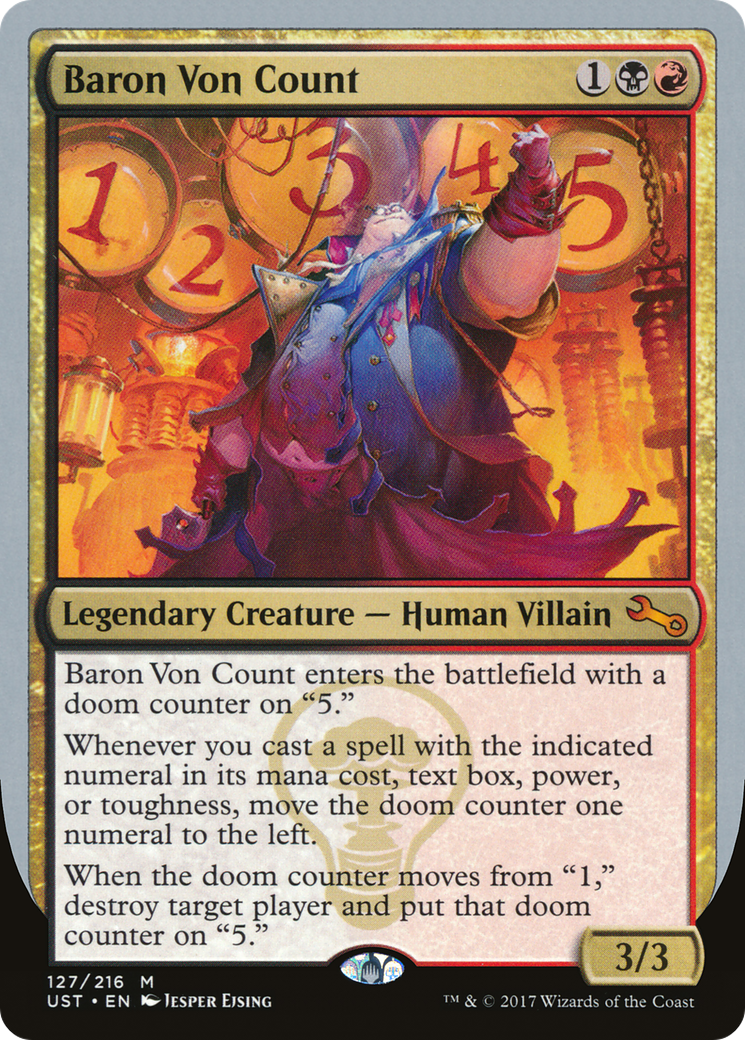Baron Von Count triggers when a player casts a spell with a certain numeral in its text box. But what exactly is a numeral? Why didn't they just say number? Well, a numeral is a specific representation of a given number; a way of writing it. Multiple numerals can all refer to the same number. It's easiest to understand this with an analogy where numerals correspond to words and numbers correspond to concepts. "Teacher", "instructor", and "educator" are three different words that refer to the same concept, while "Twelve", "12", and "XII" are three different numerals that refer to the same number. Numerals don't have to be a single word either. "2⋅6" is another numeral that's also a perfectly good representation of the same number, as is "the number of months in a year on the Gregorian calendar".

Ok, so what's a digit? In this analogy, digits would correspond to letters. "12" is a single numeral that's composed of two digits, just like "teacher" is a single word composed of seven letters. Digits only exist in positional notation systems of writing numbers though. The numeral "twelve" doesn't contain any digits, nor does "XII". (The analogous statement is true of some languages too. Japanese for example doesn't have an alphabet, it has a syllabary. Written words are composed of syllabograms rather than letters. Japanese also uses some Chinese characters, which are themselves a third type of character called a logogram. Languages are complicated.)

Just like languages, various areas of the world developed many different numeral systems throughout history to represent numbers. The modern world has mostly standardized on the Hindu–Arabic numerals, but other systems like Roman numerals and Hebrew numerals are still sometimes used for historical or aesthetic purposes.

Getting back to Baron Von Count, if we look at its rulings, we can see some that say "A numeral is any digit, such as 2, 7, or 0. Written number words such as 'five' don’t count." and "Some numbers are made up of multiple numerals. For example, if you cast a spell with the number 12 in its text box, the middle ability will trigger if the doom counter is on 1 or 2." So it looks like Wizards didn't actually know what the word "numeral" means, and just assumed that it's a synonym of "digit". Oops.

#### Set Theory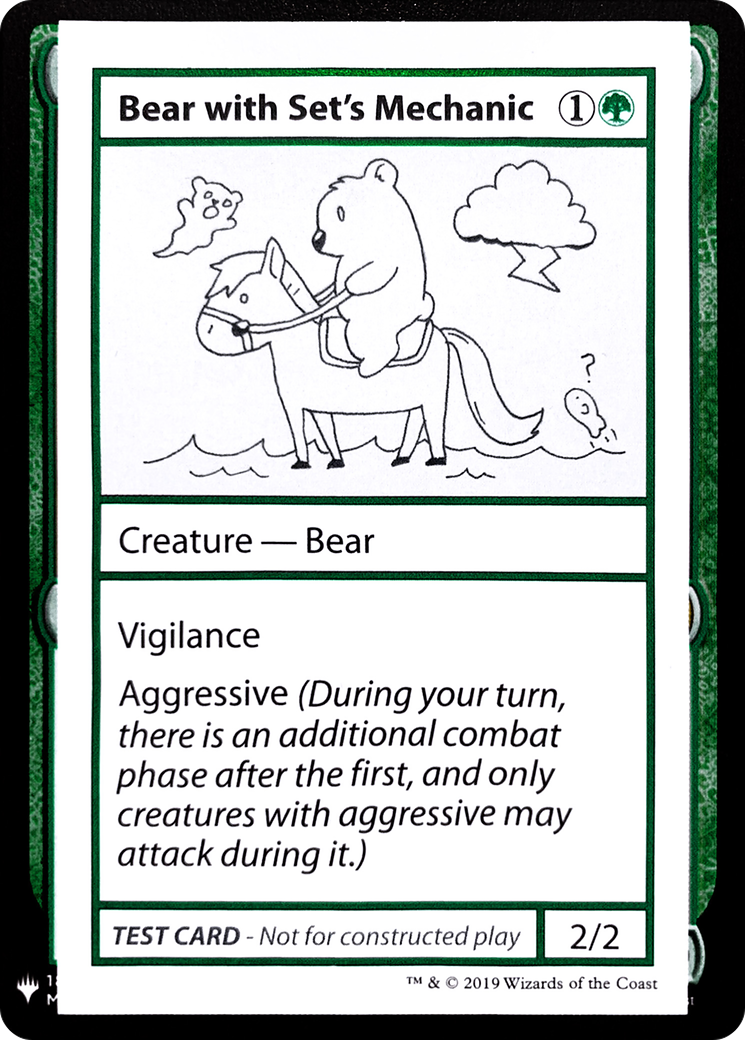Flying means that the only legal blockers are those with flying or reach. Horsemanship means that the only legal blockers are those with horsemanship. So what happens if I attack with a creature that has both flying and horsemanship? Well, both of these are restrictions on the legal blockers, and both of them can be followed, so the result is that only creatures with either "horsemanship and flying" or "horsemansip and reach" can block.

This is an example of set theory. In mathematics, a "set" is just a collection of objects or concepts. We could talk about the set of all letters in the English alphabet, the set of all Magic cards, the set of all real numbers, etc. These sets can have various relationships with each other, and we can perform operations on these sets. For example, let's take the set of all Magic cards, which currently has about 22,000 elements. Now let's take the set of all blue Magic cards. We can subtract the second from the first, and end up with the set of all nonblue Magic cards.

When it comes to evasion abilities, the overall statement of how they work is that each evasion ability defines a set of legal blockers. Flying defines the set "creatures with flying or reach", horsemanship defines the set "creatures with horsemanship", etc. Only a creature that's in all of those sets is a legal blocker. This operation is known as taking the intersection of two or more sets. For example, the intersection of the set of {Tarmogoyf, Storm Crow, Chimney Imp} and the set {Brainstorm, Counterspell, Storm Crow, Ancestral Recall} is {Storm Crow}.

Why is this useful? Let's take a look at a less intuitive demonstration of this in action. Auras each have an enchant ability that defines what it can enchant. But that statement alone is ambiguous, because what happens if an Aura gains two different enchant abilities? (Figuring out how this can occur is left as an exercise for the reader.) Can it enchant either? Both? Neither? Luckily, the comprehensive rules do define this more clearly. 702.5a makes it clear that enchant abilities restrict what an aura can enchant, just like how evasion abilities restrict what creatures can block. An Aura with both "enchant creature" and "enchant land" will only be able to enchant objects that satisfy both criteria.

Now what happens if an Aura loses all of its enchant abilities? We know that the "enchant" keyword is restrictive rather than permissive, so Auras by default can legally enchant anything. Normally there will be an enchant ability to restrict it to a smaller set, but in this case there isn't, so an Aura with no enchant abilities can enchant anything. And of course this is exactly the same as why a creature with no evasion abilities can be blocked by any creature.

This is related to what's known as a vacuous truth. A statement like "all X are Y" is always true if there are no X, regardless of what Y is. For example, the statement "all Giraffes in Magic are 2/2s" is true. This can be understood by noticing that in order for that statement to be false, its negation "at least one Giraffe in Magic has a P/T other than 2/2" must be true. There aren't any Giraffes in Magic, so it can't be true that one of them has some P/T. But we can also see how this follows from the examples around enchant and evasion abilities. An Aura has to satisfy all of its enchant abilities. If it has no enchant abilities, it is satisfying all of them.

That's all I had room for in this article. If people like this sort of content, I'll post a part 2 with some more concepts that didn't make the cut this time. I'm eager to hear whether you find this sort of thing interesting, so please leave your thoughts in the comments.

And if you're looking for place to share this sort of discussion with like-minded individuals, come join us in the RulesGuru discord server.